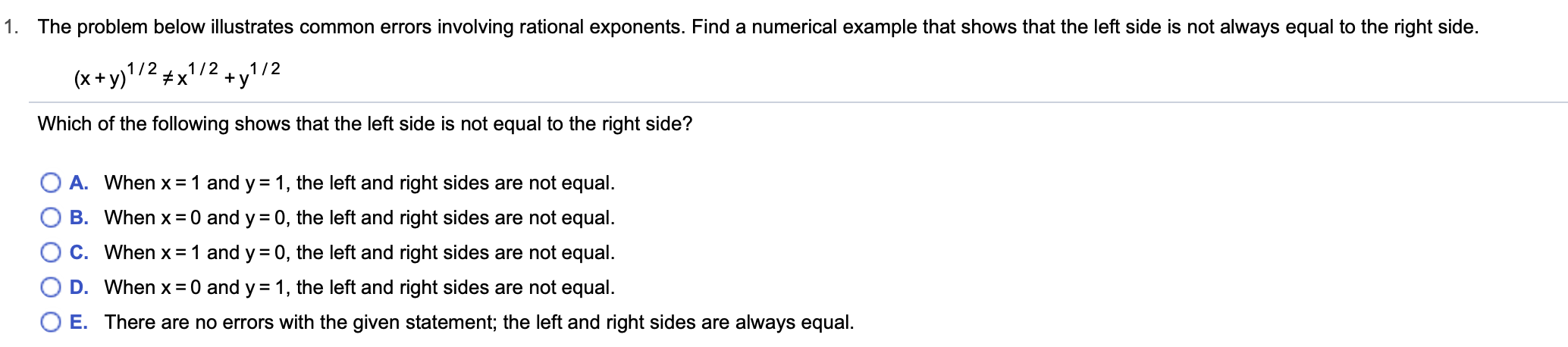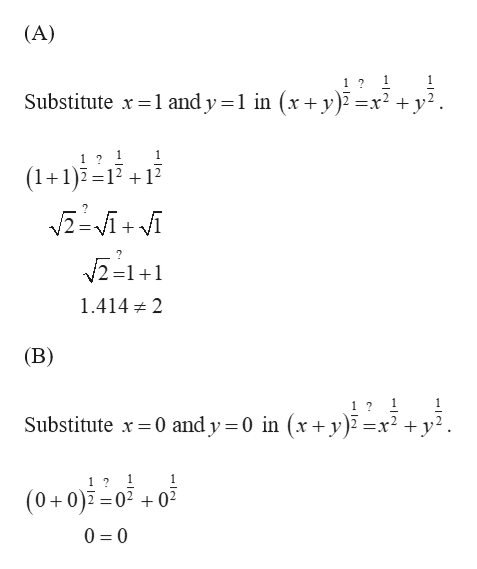# 1. The problem below illustrates common errors involving rational exponents. Find a numerical example that shows that the left side is not always equal to the right side.1/ 21/2x(x+y)1/2yWhich of the following shows that the left side is not equal to the right side?A. When x 1 and y = 1, the left and right sides are not equalB. When x 0 and y 0, the left and right sides are not equalC. When x 1 and y 0, the left and right sides are not equal.D. When x 0 and y 1, the left and right sides are not equal.O E. There are no errors with the given statement; the left and right sides are alwayss equal

Question
10 views

Can you help with this problem step by step?help_outlineImage Transcriptionclose1. The problem below illustrates common errors involving rational exponents. Find a numerical example that shows that the left side is not always equal to the right side. 1/ 21/2 x (x+y)1/2 y Which of the following shows that the left side is not equal to the right side? A. When x 1 and y = 1, the left and right sides are not equal B. When x 0 and y 0, the left and right sides are not equal C. When x 1 and y 0, the left and right sides are not equal. D. When x 0 and y 1, the left and right sides are not equal. O E. There are no errors with the given statement; the left and right sides are alwayss equal fullscreen
check_circle

Step 1help_outlineImage Transcriptionclose(A) 1 Substitute x 1 and y 1 in (x + y) x y = 1 1? 1 (1+1) =12 12 /2 =1+1 1.4142 (B) 1 Substitute 0 and y 0 in (x+ y)2 =: =x2 y 1 1 2 (0+0)0 020 0 0 fullscreen

### Want to see the full answer?

See Solution

#### Want to see this answer and more?

Solutions are written by subject experts who are available 24/7. Questions are typically answered within 1 hour.*

See Solution
*Response times may vary by subject and question.
Tagged in

### Other